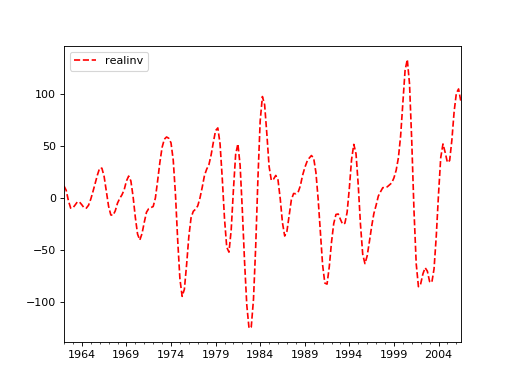# statsmodels.tsa.filters.bk_filter.bkfilter¶

statsmodels.tsa.filters.bk_filter.bkfilter(X, low=6, high=32, K=12)[source]

Baxter-King bandpass filter

Parameters
Xarray-like

A 1 or 2d ndarray. If 2d, variables are assumed to be in columns.

lowfloat

Minimum period for oscillations, ie., Baxter and King suggest that the Burns-Mitchell U.S. business cycle has 6 for quarterly data and 1.5 for annual data.

highfloat

Maximum period for oscillations BK suggest that the U.S. business cycle has 32 for quarterly data and 8 for annual data.

Kint

Lead-lag length of the filter. Baxter and King propose a truncation length of 12 for quarterly data and 3 for annual data.

Returns
Yarray

Cyclical component of X

Notes

Returns a centered weighted moving average of the original series. Where the weights a[j] are computed

a[j] = b[j] + theta, for j = 0, +/-1, +/-2, ... +/- K
b = (omega_2 - omega_1)/pi
b[j] = 1/(pi*j)(sin(omega_2*j)-sin(omega_1*j), for j = +/-1, +/-2,...


and theta is a normalizing constant

theta = -sum(b)/(2K+1)


References

Baxter, M. and R. G. King. “Measuring Business Cycles: Approximate

Band-Pass Filters for Economic Time Series.” Review of Economics and Statistics, 1999, 81(4), 575-593.

Examples

>>> import statsmodels.api as sm
>>> import pandas as pd

>>> cycles = sm.tsa.filters.bkfilter(dta[['realinv']], 6, 24, 12)

>>> import matplotlib.pyplot as plt